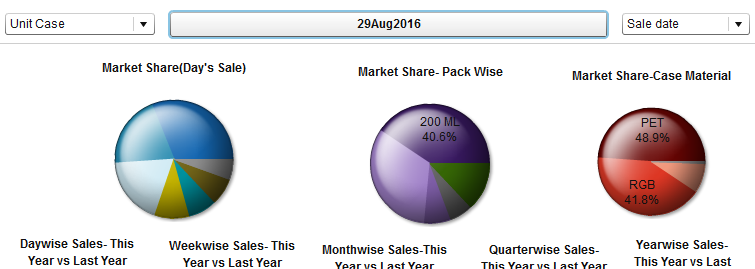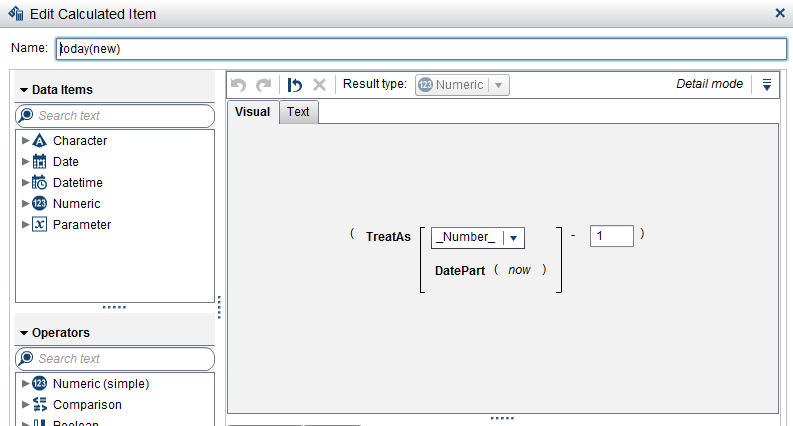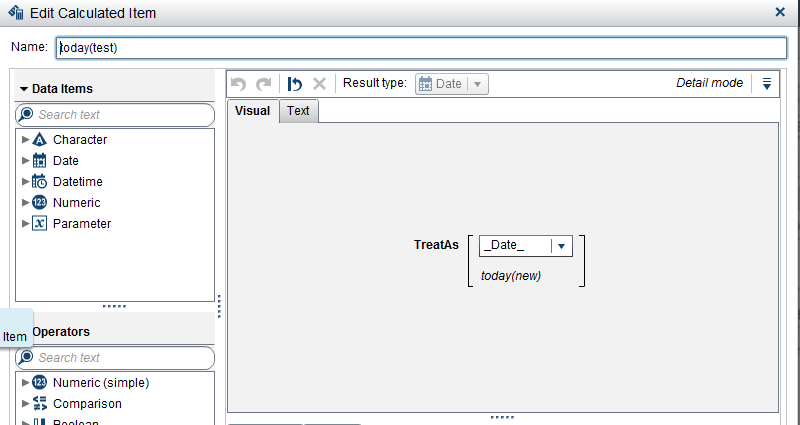## How to Create A DATE Button IN VA which can simply Let you SEE the DATA for Today

Hi.

I am supposed to create a Button for Customer which can auto refresh the entire DATA based on the present DAY.

Something like stated in the picture below.This can easily be done with the NOW() function but now the issues is the data should be less on day from the present Day. i.e. in this case 28th August 2016.

How to achieve this?

how to get a date less than the present Date.

1 ACCEPTED SOLUTION

Accepted Solutions

## Re: How to Create A DATE Button IN VA which can simply Let you SEE the DATA for Today

So I found the Answer Myself!!!

Step I:    Create a Now() field from the data.This would auto populate today's Date.

Step II: Create a new calcualted Item.Using Treat as fucntion and Datepart function change the Now data field to Number and Subtract the number of days you want from it as shown below:Step III: USing this calucated Item crete another calculated item and again use this numeric data to chage into date itme for this time as shown below:## Re: How to Create A DATE Button IN VA which can simply Let you SEE the DATA for Today

So I found the Answer Myself!!!

Step I:    Create a Now() field from the data.This would auto populate today's Date.

Step II: Create a new calcualted Item.Using Treat as fucntion and Datepart function change the Now data field to Number and Subtract the number of days you want from it as shown below:Step III: USing this calucated Item crete another calculated item and again use this numeric data to chage into date itme for this time as shown below:Discussion stats